• pytorch学习笔记（十四）————正则化惩罚（减轻overfitting）目录回顾降低过拟合方法正则化惩罚项常用的正则化公式 目录 回顾 在上一篇博客中我们讲到，当训练模型比真实模型复杂度低的情况叫做underfitting（欠...
• overfitting

2019-06-24 14:54:38
机器学习的效果如何得看他的泛化能力咋样，学的不咋的，又可以分为...overfitting，即在看多的训练集上表现的很好，计算的hypothesis完全符合看过的数据点，但是Eout不好。与underfitting不同，overfitting的成因很...

机器学习的效果如何得看他的泛化能力咋样，学的不咋的，又可以分为：underfitting，即在看过的数据集上就没有做好，这时候可以增加hypothesis 模型复杂度，通过feature transform增加dvc。overfitting，即在看多的训练集上表现的很好，计算的hypothesis完全符合看过的数据点，但是Eout不好。与underfitting不同，overfitting的成因很多，因此可使用改进的方法也很多！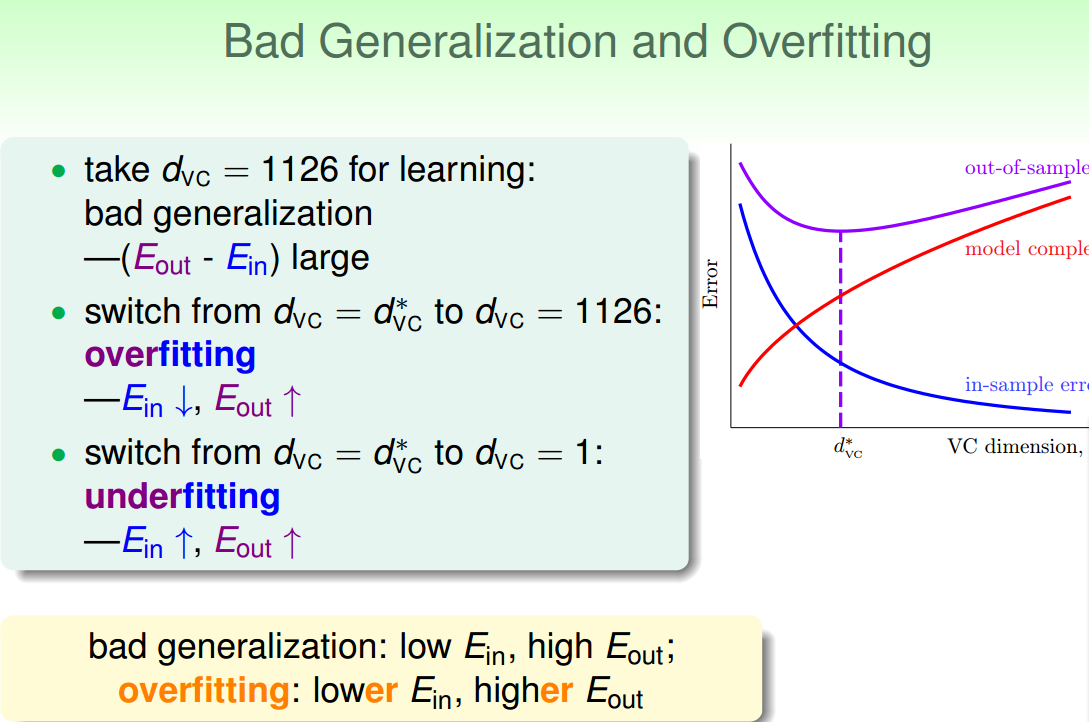一、overfitting 成因
先来看一个奇怪的现象：即便目标函数是高阶的，使用一个高阶的hypothesis学习效果却不如低阶hypothesis，这里的原因是数据点太少了，无法刻画出真实的hypothesis变化。数据集不够大，低阶的hypothesis反而更好！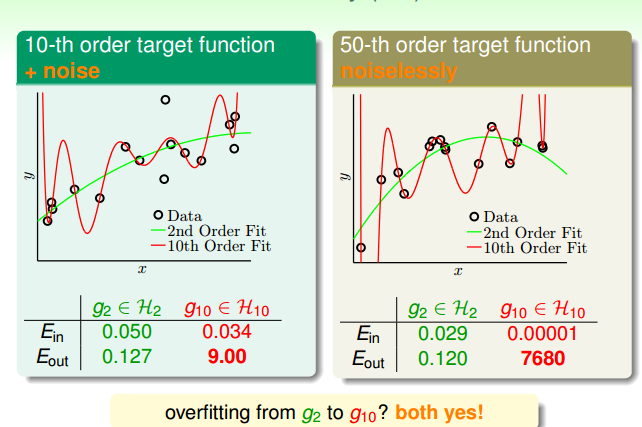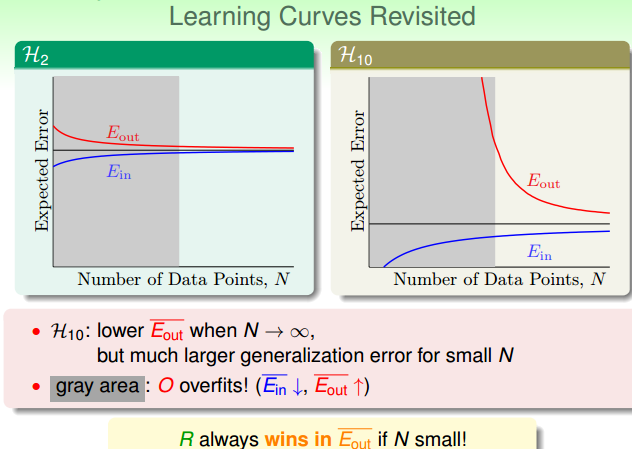假设数据通过目标函数和噪声产生，考虑噪声、目标函数复杂性、数据集大小对泛化能力影响：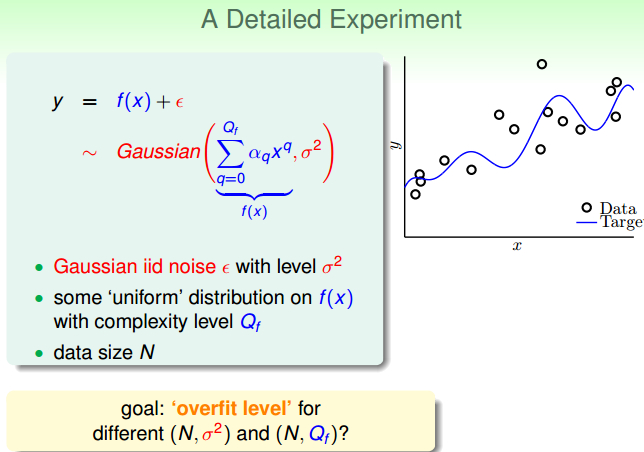选取二阶和十阶的hypothesis，先判断数据集大小和噪声强弱对泛化能力的影响，已知目标函数为20阶，衡量标准为10阶多项式和2阶多项式误差差值：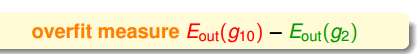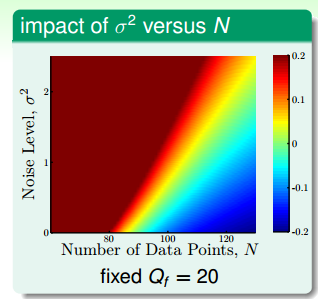判断目标函数的复杂度（样本数据跳跃性更大仿佛有噪声干扰）和数据集大小对泛化能力影响，这里的衡量标准依旧是标准为10阶多项式和2阶多项式误差差值：在最下面，此时目标函数的复杂性不高，但是使用10阶多项式易产生过拟合，与上面的分析类似，当数据量少，目标函数复杂度高时，均易产生过拟合：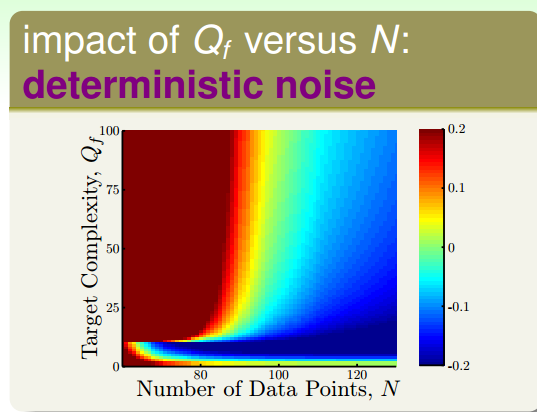总结来说：数据量少，采取hypothesis模型复杂度过高，目标函数很复杂时，均易产生过拟合！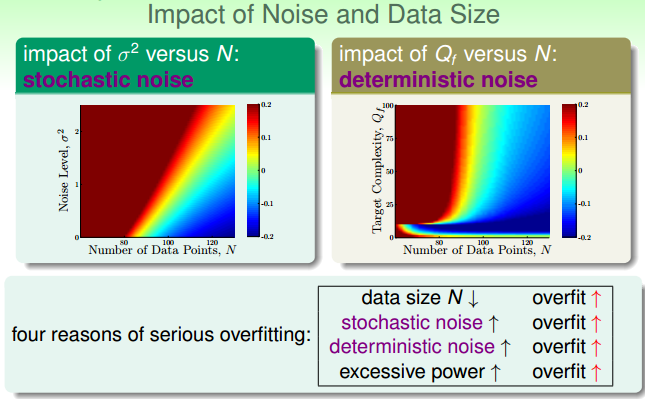deterministic Noise是最优hypothesis和target function之间的差距（图中灰色部分），determinstic Noise 总是存在的，因为hypothesis 不可能完美的拟合target function。为了缩小deterministic，当我们得知target不是那么复杂时，选用的hypothesis 也应该尽可能简单。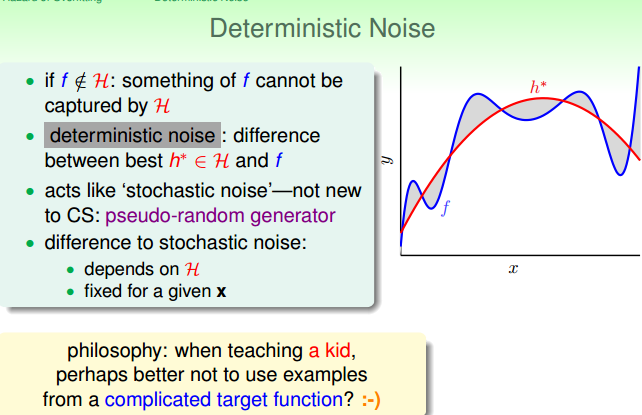二、如何解决overfitting
避免hypothesis set的vc dimension过大，那么从简单的hypothesis set开始做起！
避免Noise 的影响，对应就有data cleaning（修正错误数据点） 或是data pruning （剔除错误数据点）！比如可利用k-means方法，找出离群点，进一步修正或是剔除！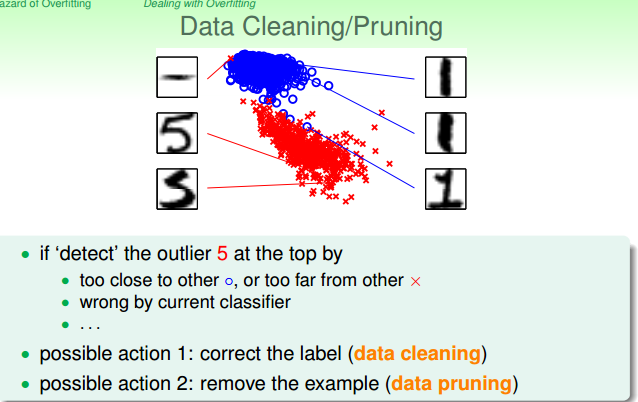避免数据量太少，可以做data hinting！利用已有的资料做一些变化（平移旋转），得到virtual example（与原有数据差别不要太大，防止破坏数据disturbtion） ！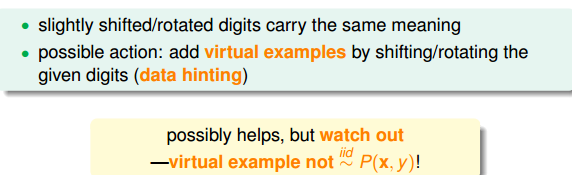当发现hypothesis 的复杂度已经过大时，可使用regularization！
以及使用validation 时刻关注拟合状况！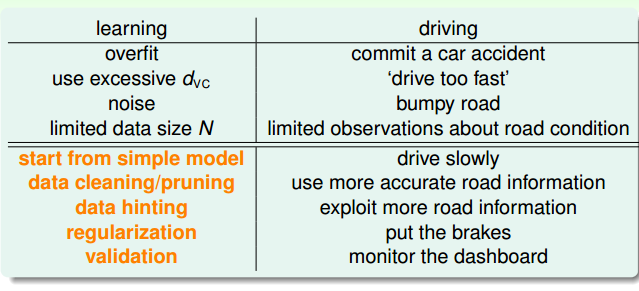总结：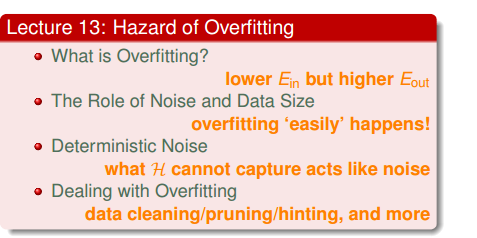展开全文• Dropout：A Simple Way to Prevent Neural Networks from Overfitting.zip深度学习
• Pytorch教程目录 Torch and Numpy 变量 (Variable) 激励函数 关系拟合（回归） 区分类型 (分类) 快速搭建法 批训练 加速神经网络训练 Optimizer优化器 ...什么是过拟合 (Overfitting) 目录Pytorch教程目

什么是过拟合 (Overfitting)

过于自负

在细说之前, 我们先用实际生活中的一个例子来比喻一下过拟合现象. 说白了, 就是机器学习模型于自信. 已经到了自负的阶段了. 那自负的坏处, 大家也知道, 就是在自己的小圈子里表现非凡, 不过在现实的大圈子里却往往处处碰壁. 所以在这个简介里, 我们把自负和过拟合画上等号.

回归分类的过拟合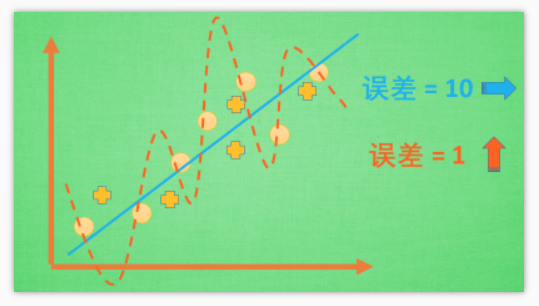机器学习模型的自负又表现在哪些方面呢. 这里是一些数据. 如果要你画一条线来描述这些数据, 大多数人都会按照蓝线这么画. 对, 这条线也是我们希望机器也能学出来的一条用来总结这些数据的线. 这时蓝线与数据的总误差可能是10.

可是有时候, 机器过于纠结这误差值, 他想把误差减到更小, 来完成他对这一批数据的学习使命. 所以, 他学到的可能会变成这样 . 它几乎经过了每一个数据点, 这样, 误差值会更小 . 可是误差越小就真的好吗? 看来我们的模型还是太天真了. 当我拿这个模型运用在现实中的时候, 他的自负就体现出来. 小二, 来一打现实数据 . 这时, 之前误差大的蓝线误差基本保持不变 .误差小的 红线误差值突然飙高 , 自负的红线再也骄傲不起来, 因为他不能成功的表达除了训练数据以外的其他数据. 这就叫做过拟合. Overfitting.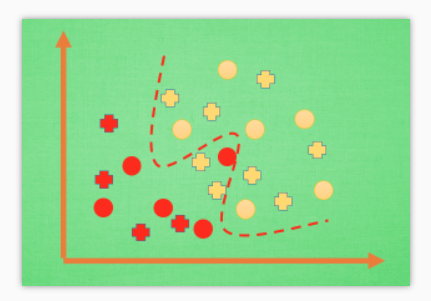那么在分类问题当中. 过拟合的分割线可能是这样, 小二, 再上一打数据 . 我们明显看出, 有两个黄色的数据并没有被很好的分隔开来. 这也是过拟合在作怪.好了, 既然我们时不时会遇到过拟合问题, 那解决的方法有那些呢.

解决方法

• 方法一: 增加数据量, 大部分过拟合产生的原因是因为数据量太少了. 如果我们有成千上万的数据, 红线也会慢慢被拉直, 变得没那么扭曲 .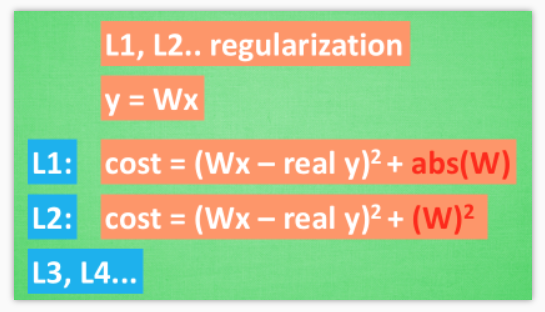• 方法二: 运用正规化. L1, L2 regularization等等, 这些方法适用于大多数的机器学习, 包括神经网络. 他们的做法大同小异, 我们简化机器学习的关键公式为 y=Wx . W为机器需要学习到的各种参数. 在过拟合中, W 的值往往变化得特别大或特别小. 为了不让W变化太大, 我们在计算误差上做些手脚.
原始的 cost 误差是这样计算, cost = 预测值-真实值的平方. 如果 W 变得太大, 我们就让 cost 也跟着变大, 变成一种惩罚机制. 所以我们把 W 自己考虑进来. 这里 abs 是绝对值. 这一种形式的 正规化, 叫做 L1 正规化.
L2 正规化和 l1 类似, 只是绝对值换成了平方. 其他的l3, l4 也都是换成了立方和4次方等等. 形式类似.
用这些方法,我们就能保证让学出来的线条不会过于扭曲.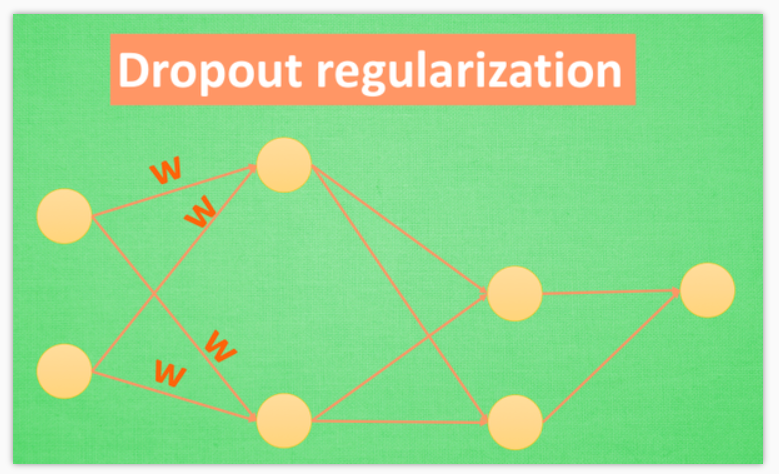• 方法三：还有一种专门用在神经网络的正规化的方法, 叫作 dropout. 在训练的时候, 我们随机忽略掉一些神经元和神经联结 , 是这个神经网络变得”不完整”. 用一个不完整的神经网络训练一次.
到第二次再随机忽略另一些, 变成另一个不完整的神经网络. 有了这些随机 drop 掉的规则, 我们可以想象其实每次训练的时候, 我们都让每一次预测结果都不会依赖于其中某部分特定的神经元. 像l1, l2正规化一样, 过度依赖的 W , 也就是训练参数的数值会很大, l1, l2会惩罚这些大的 参数. Dropout 的做法是从根本上让神经网络没机会过度依赖.

Dropout 缓解过拟合

做点数据

自己做一些伪数据, 用来模拟真实情况. 数据少, 才能凸显过拟合问题, 所以我们就做10个数据点.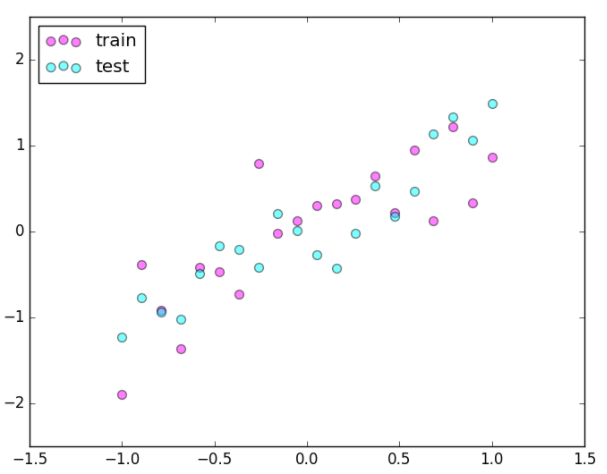import torch
import matplotlib.pyplot as plt

torch.manual_seed(1)    # reproducible

N_SAMPLES = 20
N_HIDDEN = 300

# training data
x = torch.unsqueeze(torch.linspace(-1, 1, N_SAMPLES), 1)
y = x + 0.3*torch.normal(torch.zeros(N_SAMPLES, 1), torch.ones(N_SAMPLES, 1))

# test data
test_x = torch.unsqueeze(torch.linspace(-1, 1, N_SAMPLES), 1)
test_y = test_x + 0.3*torch.normal(torch.zeros(N_SAMPLES, 1), torch.ones(N_SAMPLES, 1))

# show data
plt.scatter(x.data.numpy(), y.data.numpy(), c='magenta', s=50, alpha=0.5, label='train')
plt.scatter(test_x.data.numpy(), test_y.data.numpy(), c='cyan', s=50, alpha=0.5, label='test')
plt.legend(loc='upper left')
plt.ylim((-2.5, 2.5))
plt.show()

搭建神经网络

我们在这里搭建两个神经网络, 一个没有 dropout, 一个有 dropout. 没有 dropout 的容易出现 过拟合, 那我们就命名为 net_overfitting, 另一个就是 net_dropped. torch.nn.Dropout(0.5) 这里的 0.5 指的是随机有 50% 的神经元会被关闭/丢弃.

net_overfitting = torch.nn.Sequential(
torch.nn.Linear(1, N_HIDDEN),
torch.nn.ReLU(),
torch.nn.Linear(N_HIDDEN, N_HIDDEN),
torch.nn.ReLU(),
torch.nn.Linear(N_HIDDEN, 1),
)

net_dropped = torch.nn.Sequential(
torch.nn.Linear(1, N_HIDDEN),
torch.nn.Dropout(0.5),  # drop 50% of the neuron
torch.nn.ReLU(),
torch.nn.Linear(N_HIDDEN, N_HIDDEN),
torch.nn.Dropout(0.5),  # drop 50% of the neuron
torch.nn.ReLU(),
torch.nn.Linear(N_HIDDEN, 1),
)

训练

训练的时候, 这两个神经网络分开训练. 训练的环境都一样.

loss_func = torch.nn.MSELoss()

for t in range(500):
pred_ofit = net_overfitting(x)
pred_drop = net_dropped(x)

loss_ofit = loss_func(pred_ofit, y)
loss_drop = loss_func(pred_drop, y)

loss_ofit.backward()
loss_drop.backward()
optimizer_ofit.step()
optimizer_drop.step()

对比测试结果

在这个 for 循环里, 我们加上画测试图的部分. 注意在测试时, 要将网络改成 eval()形式, 特别是 net_dropped, net_overfitting 改不改其实无所谓. 画好图再改回 train() 模式....

optimizer_ofit.step()
optimizer_drop.step()

# 接着上面来
if t % 10 == 0:     # 每 10 步画一次图
# 将神经网络转换成测试形式, 画好图之后改回 训练形式
net_overfitting.eval()
net_dropped.eval()  # 因为 drop 网络在 train 的时候和 test 的时候参数不一样.

# plotting
plt.cla()
test_pred_ofit = net_overfitting(test_x)
test_pred_drop = net_dropped(test_x)
plt.scatter(x.data.numpy(), y.data.numpy(), c='magenta', s=50, alpha=0.3, label='train')
plt.scatter(test_x.data.numpy(), test_y.data.numpy(), c='cyan', s=50, alpha=0.3, label='test')
plt.plot(test_x.data.numpy(), test_pred_ofit.data.numpy(), 'r-', lw=3, label='overfitting')
plt.plot(test_x.data.numpy(), test_pred_drop.data.numpy(), 'b--', lw=3, label='dropout(50%)')
plt.text(0, -1.2, 'overfitting loss=%.4f' % loss_func(test_pred_ofit, test_y).data.numpy(), fontdict={'size': 20, 'color':  'red'})
plt.text(0, -1.5, 'dropout loss=%.4f' % loss_func(test_pred_drop, test_y).data.numpy(), fontdict={'size': 20, 'color': 'blue'})
plt.legend(loc='upper left'); plt.ylim((-2.5, 2.5));plt.pause(0.1)

# 将两个网络改回 训练形式
net_overfitting.train()
net_dropped.train()

plt.ioff()
plt.show()

全部代码

import torch
import matplotlib.pyplot as plt

# torch.manual_seed(1)    # reproducible

N_SAMPLES = 20
N_HIDDEN = 300

# training data
x = torch.unsqueeze(torch.linspace(-1, 1, N_SAMPLES), 1)
y = x + 0.3*torch.normal(torch.zeros(N_SAMPLES, 1), torch.ones(N_SAMPLES, 1))

# test data
test_x = torch.unsqueeze(torch.linspace(-1, 1, N_SAMPLES), 1)
test_y = test_x + 0.3*torch.normal(torch.zeros(N_SAMPLES, 1), torch.ones(N_SAMPLES, 1))

# show data
plt.scatter(x.data.numpy(), y.data.numpy(), c='magenta', s=50, alpha=0.5, label='train')
plt.scatter(test_x.data.numpy(), test_y.data.numpy(), c='cyan', s=50, alpha=0.5, label='test')
plt.legend(loc='upper left')
plt.ylim((-2.5, 2.5))
plt.show()

net_overfitting = torch.nn.Sequential(
torch.nn.Linear(1, N_HIDDEN),
torch.nn.ReLU(),
torch.nn.Linear(N_HIDDEN, N_HIDDEN),
torch.nn.ReLU(),
torch.nn.Linear(N_HIDDEN, 1),
)

net_dropped = torch.nn.Sequential(
torch.nn.Linear(1, N_HIDDEN),
torch.nn.Dropout(0.5),  # drop 50% of the neuron
torch.nn.ReLU(),
torch.nn.Linear(N_HIDDEN, N_HIDDEN),
torch.nn.Dropout(0.5),  # drop 50% of the neuron
torch.nn.ReLU(),
torch.nn.Linear(N_HIDDEN, 1),
)

print(net_overfitting)  # net architecture
print(net_dropped)

loss_func = torch.nn.MSELoss()

for t in range(500):
pred_ofit = net_overfitting(x)
pred_drop = net_dropped(x)
loss_ofit = loss_func(pred_ofit, y)
loss_drop = loss_func(pred_drop, y)

loss_ofit.backward()
loss_drop.backward()
optimizer_ofit.step()
optimizer_drop.step()

if t % 10 == 0:
# change to eval mode in order to fix drop out effect
net_overfitting.eval()
net_dropped.eval()  # parameters for dropout differ from train mode

# plotting
plt.cla()
test_pred_ofit = net_overfitting(test_x)
test_pred_drop = net_dropped(test_x)
plt.scatter(x.data.numpy(), y.data.numpy(), c='magenta', s=50, alpha=0.3, label='train')
plt.scatter(test_x.data.numpy(), test_y.data.numpy(), c='cyan', s=50, alpha=0.3, label='test')
plt.plot(test_x.data.numpy(), test_pred_ofit.data.numpy(), 'r-', lw=3, label='overfitting')
plt.plot(test_x.data.numpy(), test_pred_drop.data.numpy(), 'b--', lw=3, label='dropout(50%)')
plt.text(0, -1.2, 'overfitting loss=%.4f' % loss_func(test_pred_ofit, test_y).data.numpy(), fontdict={'size': 20, 'color':  'red'})
plt.text(0, -1.5, 'dropout loss=%.4f' % loss_func(test_pred_drop, test_y).data.numpy(), fontdict={'size': 20, 'color': 'blue'})
plt.legend(loc='upper left'); plt.ylim((-2.5, 2.5));plt.pause(0.1)

# change back to train mode
net_overfitting.train()
net_dropped.train()

plt.ioff()
plt.show()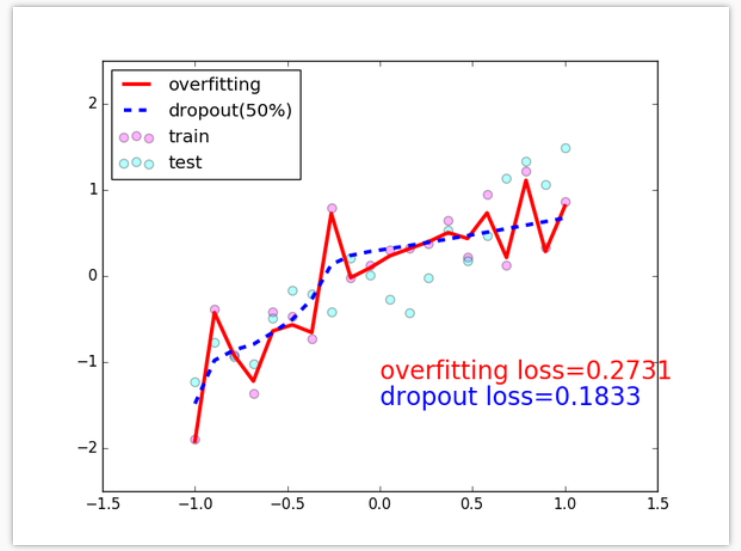展开全文• 可以通过适当学习的平滑方法来缓解稳健的过度拟合 本文的代码 陈天龙*，张振宇*，刘思佳，常世宇，王章阳 概述 为了缓解健壮的过度拟合的有趣问题，我们研究了两种在对抗训练（ AT ）中注入更多学习平滑的经验方法...
• 解决overfitting的方法

千次阅读 2018-08-27 09:29:39
解决overfitting的方法    Dropout， regularization， batch normalizatin。   但是要注意dropout只在训练的时候用，让一部分神经元随机失活。    Batch normalization是为了让输出都是单位高斯激活，...

解决overfitting的方法

Dropout， regularization， batch normalizatin。

但是要注意dropout只在训练的时候用，让一部分神经元随机失活。

Batch normalization是为了让输出都是单位高斯激活，方法是在连接和激活函数之间加入Batch Norm层，计算每个特征的均值和方差进行规则化。

展开全文• overfitting与underfitting的个人思考 bias 模型预测的平均值和真实值之间的误差。bias高，则意味着模型没有很好的拟合数据集。 varience 每次模型单独预测的值和模型多次预测的平均值之间的误差。varience高，则...

bias

模型预测的平均值和真实值之间的误差。bias高，则意味着模型没有很好的拟合数据集。

varience

每次模型单独预测的值和模型多次预测的平均值之间的误差。varience高，则意味着模型泛化能力差。

underfitting & overfitting

我们可以将一个模型看成一个杯子，这个杯子的大小由模型的参数量决定，参数量大，则杯子大；参数量小，则容器小。而数据量则是水。
当参数量小，也就是杯子小（相对于水的量），水溢出来了，这就是说明这个杯子（overfitting），即模型的varience增大（可以理解为，这个杯子只是在这个水量比较合适，当数据量改变，就不合适了）。当参数大，也就是杯子大（相对于水），水都装不满，说明这个杯子（underfitting），即模型bias增大（可以理解为，这个杯子并不是很好拟合这些水量，有更好的杯子去盛它）。
机器学习的目的就是训练出在某个问题上泛化能力强的模型

参考

展开全文• 来源于莫烦Python：过拟合 (Overfitting) 过拟合问题的通俗理解 过拟合问题相当于机器学习的模型拟合过于 “自负” 回归分类的过拟合 机器学习模型的自负又表现在哪些方面呢。这里是一些数据. 如果要你画一条线来...过拟合 解决方法
• Cat vs. Dog Image ...Exercise 2: Reducing Overfitting Estimated completion time: 30 minutes In this notebook we will build on the model we created in Exercise 1 to classify cats vs. dogs, ...
• 拟合曲面重构This paper proposes a general framework for overfitting control in surface reconstruction from noisy point data.The problem we deal with is how to create a model that will capture as much ...表面重建
• 蓝色：一元四次方程， 可以表达数据中所有的点， 但过于复杂，并且无法达到真实的数据预测， 最下面一个点可能是较大的人为误差，或则记录数据错误， 并不能反映真实的数据模型， 叫做overfitting。 绿色：虽然无法...
• overfitting problem &underfitting problem, ti's just not even fitting the training data very well. Overfitting----------- a technique called regularization that will allow us to amelioratr or to...人工智能
• 来源于莫烦Python：Dropout 解决 overfitting 往期回顾 过拟合 (Overfitting)及其解决方法 栗子    图中黑色曲线是正常模型，绿色曲线就是overfitting模型。尽管绿色曲线很精确的区分了所有的训练数据，但是并...Tensorflow Dropout
• 机器学习中的“过拟合（Overfitting）”和“欠拟合（Underfitting）” 在机器学习领域中，当讨论一个机器学习模型学习和泛化的好坏时，通常使用术语是：过拟合（Overfitting）和欠拟合（Underfitting）。过拟合和欠...
• 下面，我们就来解决overfitting（过拟合）的问题 1.Regularization （正则化） 我们先回顾一下逻辑回归中介绍的 L2 regularization，其表达式为： 还有一种 L1 regularization 方法，表达式为： 与L2 ...
• 如何防止过拟合(overfitting)

万次阅读 多人点赞 2018-09-30 10:41:32
为了得到一致假设而使假设变得过度复杂称为过拟合(overfitting)，过拟合表现在训练好的模型在训练集上效果很好，但是在测试集上效果差。也就是说模型的泛化能力弱。在很多问题中，我们费心费力收集到的数据集并不能...过拟合 Dropout
• Generalization: Peril of Overfitting  Low loss, but still a bad model? How Do We Know If Our Model Is Good? The ML Fine Print Three basic assumptions  Summary Glossay  Training and Test ...
• 然后在深度学习中又见overfitting，这下就下定决心去学习一下！！！哈哈，多学点总不会错的 step1.明白什么是overfittingoverfitting是一种现象，其对应有解决方法，主要有：①增加数据量（可以通过交叉验证...
• 在对抗性强的深度学习中过度拟合 实施实验以探究鲁棒过拟合现象的存储库，其中过强的测试性能会因训练而明显下降。由 ， 和。请在查看有关arXiv的论文。 消息 月10日-Croce 的AutoAttack框架使用此存储库评估了我们...Python机器学习 algorithm
• 缓解过拟合(overfitting)的方法

千次阅读 2020-03-15 20:54:06
为了得到一致假设而使假设变得过度复杂称为过拟合(overfitting)，过拟合表现在训练好的模型在训练集上效果很好，但是在测试集上效果差。也就是说模型的泛化能力弱。在很多问题中，我们费心费力收集到的数据集并不能...
• Dropout: A Simple Way to Prevent Neural Networks from Overfitting 原文链接：Dropout: A Simple Way to Prevent Neural Networks from Overfitting 来填坑了，上次读了《Deep Learning under Privileged ...
• We use the regulizaiton to limit the feasible region to prevent from overfitting the λ controls the limitation of the feasible region   L1 and L2 Regulization L1 norm could help use ...
• 机器学习（八）- Overfitting

千次阅读 2017-07-19 10:16:08
Overfitting问题 什么是overfittingoverfitting这个词的字面上看来就是过度拟合，我们知道的，不管什么东西，过度了一般都是不好的。 overfitting：如果我们选取了太多太多的特征，那么通过学习的模型将会...过拟合
• **方差(Variance)**代表过拟合(Overfitting)，即训练集误差很小，但验证集误差很大。 high Variance high bias high bias & high variance(欠拟合) low bias & low variance (过拟合) Training Set...
• how well the model explains the total variation in the data), we also want to avoid overfitting so that our model honestly reflects the real trend in the data. Comparing R a d j 2 R_{adj}^2 Radj2​ ...统计学
• 过拟合表现:在训练数据上的误差非常小，而在测试数据上误差反而增大。其原因一般是模型过于复杂，过分得去拟合数据的噪声和outliers. 正则化则是对模型参数添加先验，使得模型复杂度较小，对于噪声以及outliers的...
• What I'd like to do in this video is explain to you what is this overfitting problem, and in the next few videos after this, we'll talk about a technique called regularization, that will allow us to ...
• 在机器学习训练模型的过程中，若其设置了较多变量而训练数据有限时，可能会造成 Overfitting（过拟合现象），解决过拟合的方法有： 减少特征数量 （1） 人工选择需要保留的特征 （2）使用模型选择算法，自动剔除...机器学习 算法...